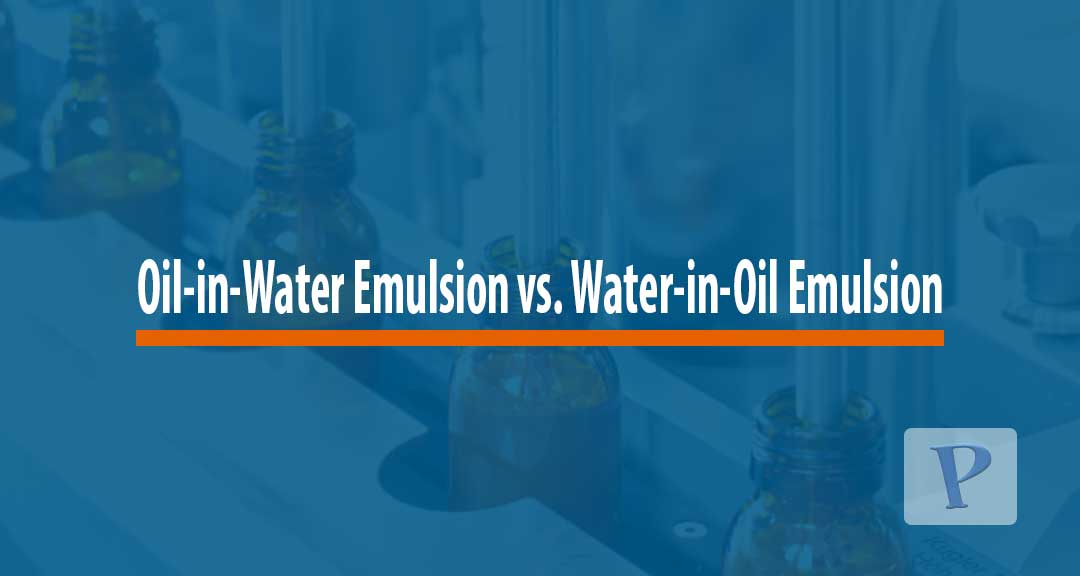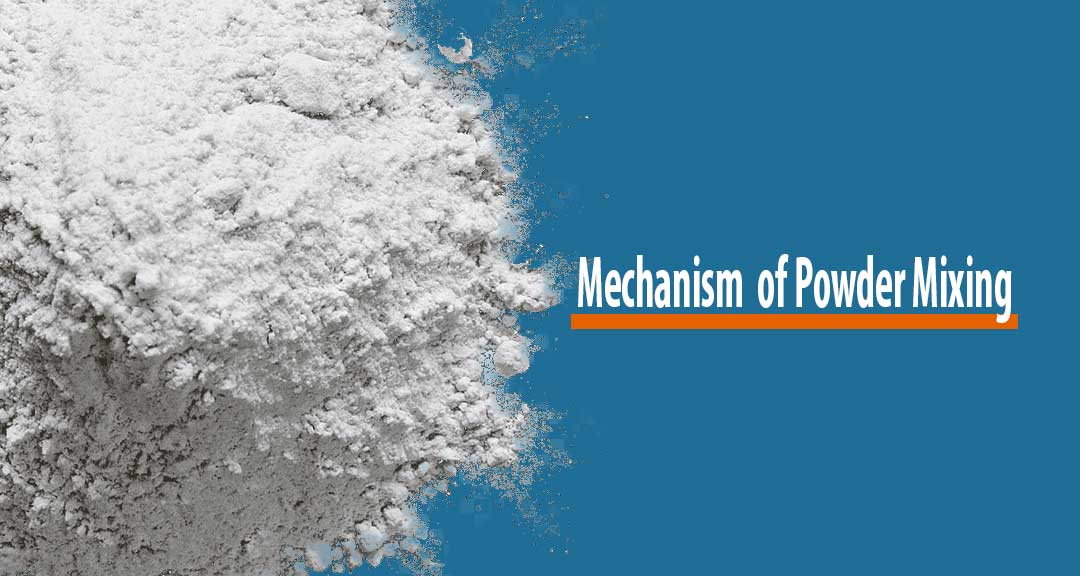# Limitations of Stokes’ Law

by | September 1, 2020

Stokes’ law is a generalized equation that describes how certain factors affect the rate of settling in dispersed systems. The implication is that, as the average particle size of suspended particles is increased, there is a dramatic effect on the resultant rate of sedimentation.

Stokes’ law is mathematically stated as

V = d21 – ρ2) g / 18 η

Where:
V = velocity of sedimentation, d = diameter of the particle, g = acceleration of gravity, ρ1 = density of the particle, ρ2 = density of the vehicle, η = viscosity of the vehicle.

Stokes’ law is based upon several assumptions, which may not always hold true for pharmaceutical suspensions. The law is valid for diluted pharmaceutical suspensions that are composed of no more than 2% solids.

In a diluted suspension, the solid particles settle without interference from one another in what is termed free settling. In a concentrated suspension, this interference may occur and may hinder the settling results.

Particle shape and size are also important in Stokes’ equation. This equation assumes spherical and monodisperse particles, which may not be encountered in real systems. Alexander et al. in 1990 introduced an equation to accommodate particles with different shapes as shown below.

V= n

where, V’ is the corrected rate of settling and ε is the initial porosity of the system, which represents the initial volume fraction of the uniformly mixed suspension. The values of ε range from zero to unity. The exponent n, which is a constant for each system, is a measure of the hindering of the system.

Limitations to Stoke’s law according to Kulshreshtha et al., include:

## 1. Negative density difference in Stoke’s equation

Stokes’ equation is invalid if the density difference in the equation is negative that is when the particles are lighter than the dispersion medium. This results in floatation or creaming most commonly seen in emulsion systems.

## 2. High content of dispersed solids

When the solid content of a suspension is high, Stokes’ equation may not show the real sedimentation rate. High solid content imparts additional viscosity to the system, which must be taken into consideration if the correct rate of settling is to be determined. The equation contains only the viscosity of the medium.

## 3. Dielectric constant

Dielectric constant ignored in Stokes’ equation is an important parameter in many situations. The electrical potential between two charges has an inverse relationship with the dielectric constant of the medium. Therefore, zeta potential is dependent on the dielectric constant of the medium.

The implication is that if a vehicle has low dielectric constant, the double layer is many times thicker than in an aqueous medium resulting also to a different zeta potential and, thus, the settling will be different. These factors are important when nonaqueous vehicles, e.g., sesame oil, corn oil, chlorofluorocarbon propellant (in aerosol suspension) are used.

## 4. Brownian movement

Brownian movement, a random (zig-zag) movement of particles suspended in a fluid resulting from their collision with the fast-moving atoms or molecules in the gas or liquid, is another factor that can influence the accuracy of the results obtained in Stokes’ equation. Brownian movement counteracts sedimentation to a measurable extent. This may lead to a great deviation of the actual rate of sedimentation from the one calculated using Stokes’ equation.

As the particle size is reduced, Brownian movement becomes significant. Below a critical radius, the movement will be sufficient to keep the particles from sedimentation. Although Stokes’ equation does not consider many important parameters, it, nevertheless, provides a very good estimate, based on what further investigation can be carried out to determine the exact rate of sedimentation.

## Reference

• Kulshreshtha A., Singh O. and Wall M. (2010). Pharmaceutical Suspensions: From Formulation Development to Manufacturing. London, New York, Dordrecht Heidelberg: Springer.

Related keywords for Limitations of Stokes’ Law include limitations of stokes law, stokes law derivation, limitations of stokes law in sedimentation analysis7 Differences between Oil-in-Water and Water-in-Oil Emulsions15 Quality Attributes for Emulsions